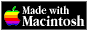THE HYPERCARD CENTER

 HyperTalk Reference
 Home » HyperTalk Reference » Functions Select a destination << Home Stack < HyperTalk Reference absannuityatanaveragecharToNumclickChunkclickH and clickVclickLineclickLocclickTextcommandKeycompoundcosdatedestinationdiskSpaceexp, exp1, exp2foundChunkfoundFieldfoundLinefoundTextheapSpacelengthln, ln1, log2maxmenusminmousemouseClickmouseH and mouseVmouseLocnumbernumToCharoffsetoptionKeyparamparamCountparamsprogramsrandomresultroundscreenRectsecondsselectedButtonselectedChunkselectedFieldselectedLineselectedLocselectedTextshiftKeysinsoundsqrtstacksstackSpacesumsystemVersiontantargettickstimetooltruncvaluewindows

# compound

`compound(number1, number2)`

where` number1 `represents the interest rate (expressed as a decimal) and` number2 `represents the number of periods over which the interest is compounded.

Value returned: value of one unit of principal invested at the interest rate and compounded over the specified number of periods, or a number equal to` (1 + rate) ^ periods`

An interest rate involves a certain percentage (expressed as a decimal) per some unit of time—usually per year. You must use the same unit of time to measure the number of periods.

For example, if you have a yearly interest rate that is compounded monthly, you must convert the yearly rate to the interest per month (`rate / 12`) and be sure to express the number of periods as months (2 years = 24 months).

## Demo Script

```on compoundDemo set numberFormat to "0.00" answer "\$100 invested for 5 years at 5.75% interest" &&¬ "compounded quarterly yields \$" & (100 * compound(.0575/4,5*4)) end compoundDemo```

## Related Topics

Version 0.7b1 (March 24, 2022)Switch to Modern View